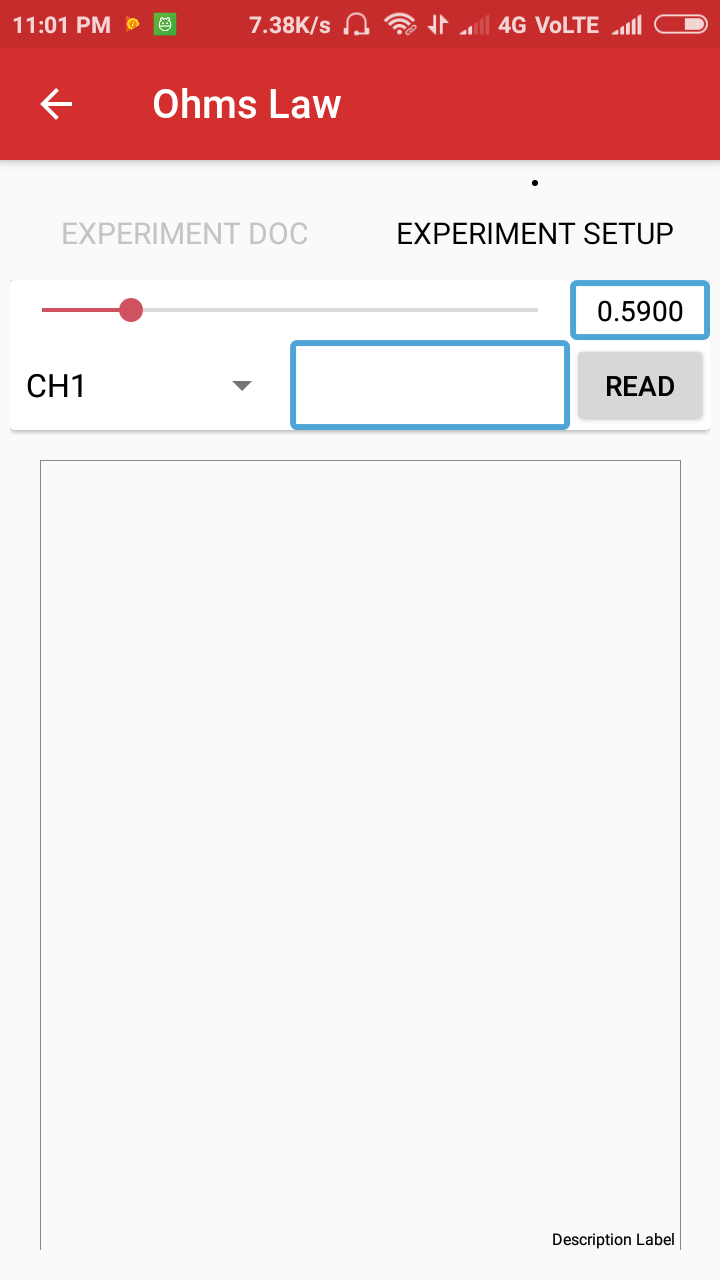# Implementing Experiment Functionality in PSLab Android

Using the PSLab Hardware Device, users can perform experiments in various domains like Electronics, Electrical, Physics, School Level experiments, etc. These experiments can be performed using functionalities exposed by hardware device like Programmable Voltage Sources, Programmable Current Source, etc. In this post we will try implementing the functionality to perform an experiment using the PSLab Hardware Device and the PSLab Android App.

Let us take the Ohm’s law experiment as an example and see how it’s implement using the  PSLab Android App.

Ohm’s law states that the current through a conductor between two points is directly proportional to the voltage across the two points, effectively using a constant of proportionality called Resistance (R) where,

`R = V / I`

#### Schematic#### Layout to perform Ohm’s law experiment

The Ohm’s law experiment requires a variable current, so a seekbar is provided to change the current coming from PCS channel, values of which are continuously reflected in the TextView next to it.#### Implementation

The Read button has a listener attached to it. Once it is clicked, the currentValue is updated with the value parsed from the seekbar progress and the selectedChannel variable is assigned from the spinner. These variables are used by the background thread to change the current supplied by current source (PCS pin) of the device and to read the updated voltage from the selected channel of the device.

```btnReadVoltage.setOnClickListener(new View.OnClickListener() {
@Override
public void onClick(View v) {
selectedChannel = channelSelectSpinner.getSelectedItem().toString();
currentValue = Double.parseDouble(tvCurrentValue.getText().toString());
if (scienceLab.isConnected()) {
CalcDataPoint calcDataPoint = new CalcDataPoint();
calcDataPoint.execute();
} else {
Toast.makeText(getContext(), "Device not connected", Toast.LENGTH_SHORT).show();
}
}
});```

CalcDataPoint is an AsyncTask which does all the underlying work like setting the current at the PCS channel, reading the voltage from the CH1 channel and triggering the update of the data points on the graph.

```private class CalcDataPoint extends AsyncTask<Void, Void, Void> {

@Override
protected Void doInBackground(Void... params) {
scienceLab.setPCS((float) currentValue);
switch (selectedChannel) {
case "CH1":
voltageValue = scienceLab.getVoltage("CH1", 5);
break;
case "CH2":
voltageValue = scienceLab.getVoltage("CH2", 5);
break;
case "CH3":
voltageValue = scienceLab.getVoltage("CH3", 5);
break;
default:
voltageValue = scienceLab.getVoltage("CH1", 5);
}
return null;
}

@Override
protected void onPostExecute(Void aVoid) {
super.onPostExecute(aVoid);
updateGraph();
}
}```

updateGraph() method is used to update the graph on UI thread. It creates a new dataset from the points which were added by the background thread and refreshes the graph with it using the invalidate() method.

```private void updateGraph() {
tvVoltageValue.setText(df.format(voltageValue));
List<ILineDataSet> dataSets = new ArrayList<>();
List<Entry> temp = new ArrayList<>();
for (int i = 0; i < x.size(); i++) {
}
LineDataSet dataSet = new LineDataSet(temp, "I-V Characteristic");
dataSet.setColor(Color.RED);
dataSet.setDrawValues(false);